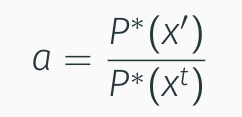# Understanding Metropolis Hasting Algorithm

In a previous post, we looked into how importance sampling works. In a sentence, importance sampling finds an estimate of the expectation of some function f under some probability distribution P by sampling from a simpler distribution Q and evaluation the expectation of the function f under Q with the terms in the sum scaled by weights obtained from the ratio unnormalized functions Q* and P*. For a more clear and detailed understanding read this post.

## Isn't importance sampling sufficient?

The issue with importance sampling is that the performance of the algorithm is highly dependant on the proposal distribution Q. The effect of a bad proposal comes into play at higher dimensions. A bad proposal could mean that we might take a long time to obtain samples from the typical set of P.

## The solution: Metropolis Hasting

The metropolis hasting method addresses this issue by using proposal distribution Q that depend on the current state. A simple example is for a given state x, the proposal can be a Gaussian distribution with mean at x and standard deviation 1. Unlike importance and rejection sampling, the generation of samples in an iteration of metropolis hasting is dependant on the sample generated in the previous iteration.

The iteration in the metropolis algorithm is as follow:

1. Propose a new sample x’ conditioned on the current sample value xᵗ using the proposal distribution Q
2. Calculate an acceptance ratio for the new proposed sample x’
3. Generate a random number in the range of 0 to 1. If the generated number is less than the calculated acceptance ratio then accept, otherwise reject.

The acceptance probability is calculated as:

In the case of symmetric proposal distributions such as the Gaussian where

the acceptance ratio takes the formFigure 3. Acceptance ratio of a new state for symmetric proposal distribution

Intuitively this suggests that the algorithm will tend to accept points that are highly likely under the target distribution P.

It can be shown that for any positive proposal function as time t, tend to infinity, the samples xᵗ will tend to the distribution P(x). In practice, however, it is often difficult to analyse if the algorithm has converged or not and how long the algorithm should be run. There are however certain rules that can be followed when employing Metropolis Hasting in higher dimensions. I will not discuss them in this post, but for the interested reader refer to chapter 24 of Kevin Murphy’s textbook.

Reference:

 Kevin Murphy, Machine Learning: A Probabilistic Perspective, Chapter 24

--

--

## More from Mohammed Suhail

Website: mohammedsuhail.net

Love podcasts or audiobooks? Learn on the go with our new app.